### scikit-learn Cookbook5 (3 reviews total)
By Trent Hauck
• FREE Subscribe Access now
• \$44.99 Print + eBook
• \$26.99 eBook
• Constantly updated with 100+ new titles each month
• Breadth and depth in over 1,000+ technologies

Python is quickly becoming the go-to language for analysts and data scientists due to its simplicity and flexibility, and within the Python data space, scikit-learn is the unequivocal choice for machine learning. Its consistent API and plethora of features help solve any machine learning problem it comes across.

The book starts by walking through different methods to prepare your data—be it a dataset with missing values or text columns that require the categories to be turned into indicator variables. After the data is ready, you'll learn different techniques aligned with different objectives—be it a dataset with known outcomes such as sales by state, or more complicated problems such as clustering similar customers. Finally, you'll learn how to polish your algorithm to ensure that it's both accurate and resilient to new datasets.

Publication date:
November 2014
Publisher
Packt
Pages
214
ISBN
9781783989485

## ChapterÂ 1.Â Premodel Workflow

This chapter will cover the following topics:

• Getting sample data from external sources

• Creating sample data for toy analysis

• Confirming the characteristics of created data

• Scaling data to the standard normal

• Creating binary features through thresholding

• Working with categorical variables

• Binarizing label features

• Imputing missing values through various strategies

• Using Pipelines for multiple preprocessing steps

• Reducing dimensionality with PCA

• Using factor analytics for decomposition

• Kernel PCA for nonlinear dimensionality reduction

• Using truncated SVD to reduce dimensionality

• Decomposition to classify with DictionaryLearning

• Putting it all together with Pipelines

• Using Gaussian processes for regression

• Defining the Gaussian process object directly

• Using stochastic gradient descent for regression

## Introduction

This chapter discusses setting data, preparing data, and premodel dimensionality reduction. These are not the attractive parts of machine learning (ML), but they often turn out to be what determines if a model will work or not.

There are three main parts to the chapter. Firstly, we'll create fake data; this might seem trivial, but creating fake data and fitting models to fake data is an important step in model testing. It's more useful in situations where we implement an algorithm from scratch, but I'll cover it here for completeness, and in the event you don't have data of your own, you can just create it. Secondly, we'll look at broadly handling data transformations as a preprocessing step, which includes data imputation, categorical variable encoding, and so on. Thirdly, we'll look at situations where we have a large number of features relative to the number of observations we have.

This chapter, especially the first half, will set the stage for the later chapters. In order to use scikit-learn, data is required. The first two sections will discuss acquiring the data; the rest of the first half will discuss preparing this data for use.

### Tip

This book is written using scikit-learn 0.15, NumPy 1.9, and pandas 0.13. There are other packages used as well, so it's advisable that you refer to the installation instructions included in this book.

## Getting sample data from external sources

If possible, try working with a familiar dataset while working through this book; in order to level the field, built-in datasets will be used. The built-in datasets can be used as stand-ins to test several different modeling techniques such as regression and classification. These are, for the most part, famous datasets. This is very useful as papers in various fields will often use these datasets for authors to put forth how their model fits as compared to other models.

### Tip

I recommend you use IPython to run these commands as they are presented. Muscle memory is important, and it's best to get to the point where basic commands take no extra mental effort. An even better way might be to run IPython Notebook. If you do, make sure to use the `%matplotlib inline` command; this will allow you to see the plots in Notebook.

The datasets in scikit-learn are contained within the `datasets` module. Use the following command to import these datasets:

```>>> from sklearn import datasets
>>> import numpy as np
```

From within IPython, run `datasets.*?`, which will list everything available within the `datasets` module.

### How to do itâ€¦

There are two main types of data within the `datasets` module. Smaller test datasets are included in the `sklearn` package and can be viewed by running `datasets.load_*?`. Larger datasets are also available for download as required. The latter are not included in `sklearn` by default; however, at times, they are better to test models and algorithms due to sufficient complexity to represent realistic situations.

Datasets are included with `sklearn` by default; to view these datasets, run `datasets.load_*?`. There are other types of datasets that must be fetched. These datasets are larger, and therefore, they do not come within the package. This said, they are often better to test algorithms that might be used in the wild.

First, load the `boston` dataset and examine it:

```>>> boston = datasets.load_boston()
>>> print boston.DESCR #output omitted due to length
```

`DESCR` will present a basic overview of the data to give you some context.

Next, fetch a dataset:

```>>> housing = datasets.fetch_california_housing()

>>> print housing.DESCR #output omitted due to length
```

### How it worksâ€¦

When these datasets are loaded, they aren't loaded as NumPy arrays. They are of type `Bunch`. A Bunch is a common data structure in Python. It's essentially a dictionary with the keys added to the object as attributes.

To access the data using the (surprise!) `data` attribute, which is a NumPy array containing the independent variables, the target attribute has the dependent variable:

```>>> X, y = boston.data, boston.target
```

There are various implementations available on the Web for the `Bunch` object; it's not too difficult to write on your own. scikit-learn defines `Bunch` (as of this writing) in the base module.

It's available in GitHub at https://github.com/scikit-learn/scikit-learn/blob/master/sklearn/datasets/base.py.

### There's moreâ€¦

When you fetch a dataset from an external source it will, by default, place the data in your home directory under `scikit_learn_data/`; this behavior is configurable in two ways:

• To modify the default behavior, set the `SCIKIT_LEARN_DATA` environment variable to point to the desired folder.

• The first argument of the fetch methods is `data_home`, which will specify the home folder on a case-by-case basis.

It is easy to check the default location by calling `datasets.get_data_home()`.

The UCI Machine Learning Repository is a great place to find sample datasets. Many of the datasets in scikit-learn are hosted here; however, there are more datasets available. Other notable sources include KDD, your local government agency, and Kaggle competitions.

## Creating sample data for toy analysis

I will again implore you to use some of your own data for this book, but in the event you cannot, we'll learn how we can use scikit-learn to create toy data.

Very similar to getting built-in datasets, fetching new datasets, and creating sample datasets, the functions that are used follow the naming convention `make_<the data set>`. Just to be clear, this data is purely artificial:

```>>> datasets.make_*?
datasets.make_biclusters
datasets.make_blobs
datasets.make_checkerboard
datasets.make_circles
datasets.make_classification
...
```

To save typing, import the `datasets` module as `d` , and `numpy` as `np`:

```>>> import sklearn.datasets as d
>>> import numpy as np
```

### How to do it...

This section will walk you through the creation of several datasets; the following How it works... section will confirm the purported characteristics of the datasets. In addition to the sample datasets, these will be used throughout the book to create data with the necessary characteristics for the algorithms on display.

First, the stalwartâ€”regression:

```>>> reg_data = d.make_regression()
```

By default, this will generate a tuple with a 100 x 100 matrix â€“ 100 samples by 100 features. However, by default, only 10 features are responsible for the target data generation. The second member of the tuple is the target variable.

It is also possible to get more involved. For example, to generate a 1000 x 10 matrix with five features responsible for the target creation, an underlying bias factor of 1.0, and 2 targets, the following command will be run:

```>>> complex_reg_data = d.make_regression(1000, 10, 5, 2, 1.0)
>>> complex_reg_data.shape
(1000, 10)
```

Classification datasets are also very simple to create. It's simple to create a base classification set, but the basic case is rarely experienced in practiceâ€”most users don't convert, most transactions aren't fraudulent, and so on. Therefore, it's useful to explore classification on unbalanced datasets:

```>>> classification_set = d.make_classification(weights=[0.1])
>>> np.bincount(classification_set)
array([10, 90])
```

Clusters will also be covered. There are actually several functions to create datasets that can be modeled by different cluster algorithms. For example, `blobs` are very easy to create and can be modeled by K-Means:

```>>> blobs = d.make_blobs()
```

This will look like the following: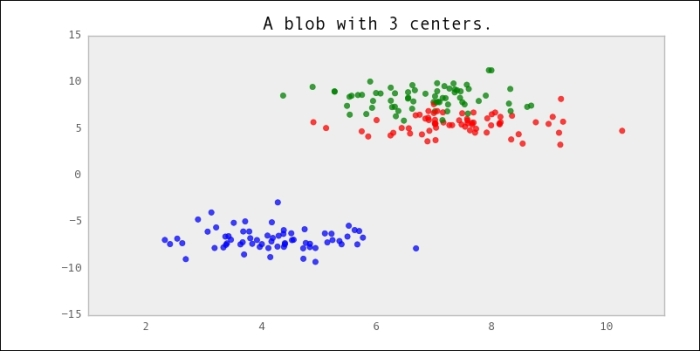### How it works...

Let's walk you through how scikit-learn produces the regression dataset by taking a look at the source code (with some modifications for clarity). Any undefined variables are assumed to have the default value of `make_regression`.

It's actually surprisingly simple to follow.

First, a random array is generated with the size specified when the function is called:

```>>> X = np.random.randn(n_samples, n_features)
```

Given the basic dataset, the target dataset is then generated:

```>>> ground_truth = np.zeroes((np_samples, n_target))
>>> ground_truth[:n_informative, :] = 100*np.random.rand(n_informative,
n_targets)
```

The dot product of `X` and `ground_truth` are taken to get the final target values. Bias, if any, is added at this time:

```>>> y = np.dot(X, ground_truth) + bias
```

### Note

The dot product is simply a matrix multiplication. So, our final dataset will have `n_samples`, which is the number of rows from the dataset, and `n_target`, which is the number of target variables.

Due to NumPy's broadcasting, bias can be a scalar value, and this value will be added to every sample.

Finally, it's a simple matter of adding any noise and shuffling the dataset. VoilÃ , we have a dataset perfect to test regression.

## Scaling data to the standard normal

A preprocessing step that is almost recommended is to scale columns to the standard normal. The standard normal is probably the most important distribution of all statistics.

If you've ever been introduced to statistics, you must have almost certainly seen z-scores. In truth, that's all this recipe is aboutâ€”transforming our features from their endowed distribution into z-scores.

The act of scaling data is extremely useful. There are a lot of machine learning algorithms, which perform differently (and incorrectly) in the event the features exist at different scales. For example, SVMs perform poorly if the data isn't scaled because it uses a distance function in its optimization, which is biased if one feature varies from 0 to 10,000 and the other varies from 0 to 1.

The `preprocessing` module contains several useful functions to scale features:

```>>> from sklearn import preprocessing
>>> import numpy as np # we'll need it later
```

### How to do it...

Continuing with the `boston` dataset, run the following commands:

```>>> X[:, :3].mean(axis=0) #mean of the first 3 features
array([  3.59376071,  11.36363636,  11.13677866])
>>> X[:, :3].std(axis=0)
array([  8.58828355,  23.29939569,   6.85357058])
```

There's actually a lot to learn from this initially. Firstly, the first feature has the smallest mean but varies even more than the third feature. The second feature has the largest mean and standard deviationâ€”it takes the widest spread of values:

```>>> X_2 = preprocessing.scale(X[:, :3])

>>> X_2.mean(axis=0)
array([  6.34099712e-17,  -6.34319123e-16,  -2.68291099e-15])

>>> X_2.std(axis=0)
array([ 1.,  1.,  1.])
```

### How it works...

The center and scaling function is extremely simple. It merely subtracts the mean and divides by the standard deviation: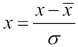In addition to a function, there is also a center and scaling class that is easy to invoke, and this is particularly useful when used in conjunction with the Pipelines mentioned later. It's also useful for the center and scaling class to persist across individual scaling:

```>>> my_scaler = preprocessing.StandardScaler()
>>> my_scaler.fit(X[:, :3])
>>> my_scaler.transform(X[:, :3]).mean(axis=0)
array([  6.34099712e-17,  -6.34319123e-16,  -2.68291099e-15])
```

Scaling features to mean `0`, and standard deviation `1` isn't the only useful type of scaling. Preprocessing also contains a `MinMaxScaler` class, which will scale the data within a certain range:

```>>> my_minmax_scaler = preprocessing.MinMaxScaler()
>>> my_minmax_scaler.fit(X[:, :3])
>>> my_minmax_scaler.transform(X[:, :3]).max(axis=0)
array([ 1.,  1.,  1.])
```

It's very simple to change the minimum and maximum values of the `MinMaxScaler` class from its default of `0` and `1`, respectively:

```>>> my_odd_scaler = preprocessing.MinMaxScaler(feature_range=(-3.14,
3.14))
```

Furthermore, another option is normalization. This will scale each sample to have a length of 1. This is different from the other types of scaling done previously, where the features were scaled. Normalization is illustrated in the following command:

```>>> normalized_X = preprocessing.normalize(X[:, :3])
```

If it's not apparent why this is useful, consider the Euclidian distance (a measure of similarity) between three of the samples, where one sample has the values (1, 1, 0), another has (3, 3, 0), and the final has (1, -1, 0).

The distance between the 1st and 3rd vector is less than the distance between the 1st and 2nd though the 1st and 3rd are orthogonal, whereas the 1st and 2nd only differ by a scalar factor of 3. Since distances are often used as measures of similarity, not normalizing the data first will be misleading..

### There's more...

Imputation is a very deep subject. Here are a few things to consider when using scikit-learn's implementation.

#### Creating idempotent scalar objects

It is possible to scale the mean and/or variance in the `StandardScaler` instance. For instance, it's possible (though not useful) to create a `StandardScaler` instance, which simply performs the identity transformation:

```>>> my_useless_scaler = preprocessing.StandardScaler(with_mean=False,
with_std=False)
>>> transformed_sd = my_useless_scaler
.fit_transform(X[:, :3]).std(axis=0)
>>> original_sd = X[:, :3].std(axis=0)
>>> np.array_equal(transformed_sd, original_sd)
```

#### Handling sparse imputations

Sparse matrices aren't handled differently from normal matrices when doing scaling. This is because to mean center the data, the data will have its 0s altered to nonzero values, thus the matrix will no longer be sparse:

```>>> matrix = scipy.sparse.eye(1000)
>>> preprocessing.scale(matrix)
â€¦
ValueError: Cannot center sparse matrices: pass 'with_mean=False' instead See docstring for motivation and alternatives.
```

As noted in the error, it is possible to scale a sparse matrix `with_std` only:

```>>> preprocessing.scale(matrix, with_mean=False)
<1000x1000 sparse matrix of type '<type 'numpy.float64'>'
with 1000 stored elements in Compressed Sparse Row format>
```

The other option is to call `todense()` on the array. However, this is dangerous because the matrix is already sparse for a reason, and it will potentially cause a memory error.

## Creating binary features through thresholding

In the last recipe, we looked at transforming our data into the standard normal distribution. Now, we'll talk about another transformation, one that is quite different.

Instead of working with the distribution to standardize it, we'll purposely throw away data; but, if we have good reason, this can be a very smart move. Often, in what is ostensibly continuous data, there are discontinuities that can be determined via binary features.

Creating binary features and outcomes is a very useful method, but it should be used with caution. Let's use the `boston` dataset to learn how to create turn values in binary outcomes.

First, load the `boston` dataset:

```>>> from sklearn import datasets
>>> import numpy as np
```

### How to do it...

Similar to scaling, there are two ways to binarize features in scikit-learn:

• `preprocessing.binarize #(a function)`

• `preprocessing.Binarizer #(a class)`

The `boston` dataset's target variable is the median value of houses in thousands. This dataset is good to test regression and other continuous predictors, but consider a situation where we want to simply predict if a house's value is more than the overall mean. To do this, we will want to create a threshold value of the mean. If the value is greater than the mean, produce a 1; if it is less, produce a 0:

```>>> from sklearn import preprocessing
>>> new_target = preprocessing.binarize(boston.target,
threshold=boston.target.mean())
>>> new_target[:5]
array([ 1.,  0.,  1.,  1.,  1.])
```

This was easy, but let's check to make sure it worked correctly:

```>>> (boston.target[:5] > boston.target.mean()).astype(int)
array([1, 0, 1, 1, 1])
```

Given the simplicity of the operation in NumPy, it's a fair question to ask why you will want to use the built-in functionality of scikit-learn. Pipelines, covered in the Using Pipelines for multiple preprocessing steps recipe, will go far to explain this; in anticipation of this, let's use the `Binarizer` class:

```>>> bin = preprocessing.Binarizer(boston.target.mean())
>>> new_target = bin.fit_transform(boston.target)
>>> new_target[:5]
array([ 1.,  0.,  1.,  1.,  1.])
```

### How it works...

Hopefully, this is pretty obvious; but under the hood, scikit-learn creates a conditional mask that is `True` if the value in the array in question is more than the threshold. It then updates the array to 1 where the condition is met, and 0 where it is not.

### There's more...

Let's also learn about sparse matrices and the `fit` method.

#### Sparse matrices

Sparse matrices are special in that zeros aren't stored; this is done in an effort to save space in memory. This creates an issue for the binarizer, so to combat it, a special condition for the binarizer for sparse matrices is that the threshold cannot be less than zero:

```>>> from scipy.sparse import coo
>>> spar = coo.coo_matrix(np.random.binomial(1, .25, 100))
>>> preprocessing.binarize(spar, threshold=-1)
ValueError: Cannot binarize a sparse matrix with threshold < 0
```

#### The fit method

The `fit` method exists for the binarizer transformation, but it will not fit anything, it will simply return the object.

## Working with categorical variables

Categorical variables are a problem. On one hand they provide valuable information; on the other hand, it's probably textâ€”either the actual text or integers corresponding to the textâ€”like an index in a lookup table.

So, we clearly need to represent our text as integers for the model's sake, but we can't just use the `id` field or naively represent them. This is because we need to avoid a similar problem to the Creating binary features through thresholding recipe. If we treat data that is continuous, it must be interpreted as continuous.

The `boston` dataset won't be useful for this section. While it's useful for feature binarization, it won't suffice for creating features from categorical variables. For this, the `iris` dataset will suffice.

For this to work, the problem needs to be turned on its head. Imagine a problem where the goal is to predict the sepal width; therefore, the species of the flower will probably be useful as a feature.

Let's get the data sorted first:

```>>> from sklearn import datasets
>>> X = iris.data
>>> y = iris.target
```

Now, with X and Y being as they normally will be, we'll operate on the data as one:

```>>> import numpy as np
>>> d = np.column_stack((X, y))
```

### How to do it...

Convert the text columns to three features:

```>>> from sklearn import preprocessing
>>> text_encoder = preprocessing.OneHotEncoder()
>>> text_encoder.fit_transform(d[:, -1:]).toarray()[:5]
array([[ 1.,  0.,  0.],
[ 1.,  0.,  0.],
[ 1.,  0.,  0.],
[ 1.,  0.,  0.],
[ 1.,  0.,  0.]])
```

### How it works...

The encoder creates additional features for each categorical variable, and the value returned is a sparse matrix. The result is a sparse matrix by definition; each row of the new features has 0 everywhere, except for the column whose value is associated with the feature's category. Therefore, it makes sense to store this data in a sparse matrix.

`text_encoder` is now a standard scikit-learn model, which means that it can be used again:

```>>> text_encoder.transform(np.ones((3, 1))).toarray()
array([[ 0.,  1.,  0.],
[ 0.,  1.,  0.],
[ 0.,  1.,  0.]])
```

### There's more...

Other options exist to create categorical variables in scikit-learn and Python at large. `DictVectorizer` is a good option if you like to limit the dependencies of your projects to only scikit-learn and you have a fairly simple encoding scheme. However, if you require more sophisticated categorical encoding, `patsy` is a very good option.

#### DictVectorizer

Another option is to use `DictVectorizer`. This can be used to directly convert strings to features:

```>>> from sklearn.feature_extraction import DictVectorizer
>>> dv = DictVectorizer()
>>> my_dict = [{'species': iris.target_names[i]} for i in y]
>>> dv.fit_transform(my_dict).toarray()[:5]
array([[ 1.,  0.,  0.],
[ 1.,  0.,  0.],
[ 1.,  0.,  0.],
[ 1.,  0.,  0.],
[ 1.,  0.,  0.]])
```

### Tip

Dictionaries can be viewed as a sparse matrix. They only contain entries for the nonzero values.

#### Patsy

`patsy` is another package useful to encode categorical variables. Often used in conjunction with `StatsModels`, `patsy` can turn an array of strings into a design matrix.

### Tip

This section does not directly pertain to scikit-learn; therefore, skipping it is okay without impacting the understanding of how scikit-learn works.

For example, `dm = patsy.design_matrix("x + y")` will create the appropriate columns if `x` or `y` are strings. If they aren't, `C(x)` inside the formula will signify that it is a categorical variable.

For example, `iris.target` can be interpreted as a continuous variable if we don't know better. Therefore, use the following command:

```>>> import patsy
>>> patsy.dmatrix("0 + C(species)", {'species': iris.target})
DesignMatrix with shape (150, 3)
C(species)  C(species)  C(species)
1              0              0
1              0              0
1              0              0
1              0              0
1              0              0
1              0              0
1              0              0
1              0              0
1              0              0
[...]
```

## Binarizing label features

In this recipe, we'll look at working with categorical variables in a different way. In the event that only one or two categories of the feature are important, it might be wise to avoid the extra dimensionality, which might be created if there are several categories.

There's another way to work with categorical variables. Instead of dealing with the categorical variables using `OneHotEncoder`, we can use `LabelBinarizer`. This is a combination of thresholding and working with categorical variables.

To show how this works, load the `iris` dataset:

```>>> from sklearn import datasets as d
>>> target = iris.target
```

### How to do it...

Import the `LabelBinarizer()` method and create an object:

```>>> from sklearn.preprocessing import LabelBinarizer
>>> label_binarizer = LabelBinarizer()
```

Now, simply transform the target outcomes to the new feature space:

```>>> new_target = label_binarizer.fit_transform(target)
```

Let's look at `new_target` and the `label_binarizer` object to get a feel of what happened:

```>>> new_target.shape
(150, 3)
>>> new_target[:5]
array([[1, 0, 0],
[1, 0, 0],
[1, 0, 0],
[1, 0, 0],
[1, 0, 0]])

>>> new_target[-5:]
array([[0, 0, 1],
[0, 0, 1],
[0, 0, 1],
[0, 0, 1],
[0, 0, 1]])

>>> label_binarizer.classes_
array([0, 1, 2])
```

### How it works...

The `iris` target has a cardinality of `3`, that is, it has three unique values. When `LabelBinarizer` converts the vector N x 1 into the vector N x C, where C is the cardinality of the N x 1 dataset, it is important to note that once the object has been fit, introducing unseen values in the transformation will throw an error:

```>>> label_binarizer.transform()
[...]
ValueError: classes [0 1 2] mismatch with the labels  found in the data
```

### There's more...

Zero and one do not have to represent the positive and negative instances of the target value. For example, if we want positive values to be represented by 1,000, and negative values to be represented by -1,000, we'd simply make the designation when we create `label_binarizer`:

```>>> label_binarizer = LabelBinarizer(neg_label=-1000, pos_label=1000)
>>> label_binarizer.fit_transform(target)[:5]
array([[ 1000, -1000, -1000],
[ 1000, -1000, -1000],
[ 1000, -1000, -1000],
[ 1000, -1000, -1000],
[ 1000, -1000, -1000]])
```

### Tip

The only restriction on the positive and negative values is that they must be integers.

## Imputing missing values through various strategies

Data imputation is critical in practice, and thankfully there are many ways to deal with it. In this recipe, we'll look at a few of the strategies. However, be aware that there might be other approaches that fit your situation better.

This means scikit-learn comes with the ability to perform fairly common imputations; it will simply apply some transformations to the existing data and fill the NAs. However, if the dataset is missing data, and there's a known reason for this missing dataâ€”for example, response times for a server that times out after 100msâ€”it might be better to take a statistical approach through other packages such as the Bayesian treatment via PyMC, the Hazard Models via Lifelines, or something home-grown.

The first thing to do to learn how to input missing values is to create missing values. NumPy's masking will make this extremely simple:

```>>> from sklearn import datasets
>>> import numpy as np
>>> iris_X = iris.data
iris_X.shape).astype(bool)
```

To unravel this a bit, in case NumPy isn't too familiar, it's possible to index arrays with other arrays in NumPy. So, to create the random missing data, a random Boolean array is created, which is of the same shape as the `iris` dataset. Then, it's possible to make an assignment via the masked array. It's important to note that because a random array is used, it is likely your `masking_array` will be different from what's used here.

To make sure this works, use the following command (since we're using a random mask, it might not match directly):

```>>> masking_array[:5]
array([[False, False, False, False],
[False, False, False, False],
[False, False, False, False],
[ True, False, False, False],
[False, False, False, False]], dtype=bool)
>>> iris_X [:5]
array([[ 5.1,  3.5,  1.4,  0.2],
[ 4.9,  3. ,  1.4,  0.2],
[ 4.7,  3.2,  1.3,  0.2],
[ nan,  3.1,  1.5,  0.2],
[ 5. ,  3.6,  1.4,  0.2]])
```

### How to do it...

A theme prevalent throughout this book (due to the theme throughout scikit-learn) is reusable classes that fit and transform datasets and that can subsequently be used to transform unseen datasets. This is illustrated as follows:

```>>> from sklearn import preprocessing
>>> impute = preprocessing.Imputer()
>>> iris_X_prime = impute.fit_transform(iris_X)
>>> iris_X_prime[:5]
array([[ 5.1       ,  3.5       ,  1.4       ,  0.2       ],
[ 4.9       ,  3.        ,  1.4       ,  0.2       ],
[ 4.7       ,  3.2       ,  1.3       ,  0.2       ],
[ 5.87923077,  3.1       ,  1.5       ,  0.2       ],
[ 5.        ,  3.6       ,  1.4       ,  0.2       ]])
```

Notice the difference in the position [3, 0]:

```>>> iris_X_prime[3, 0]
5.87923077
>>> iris_X[3, 0]
nan
```

### How it works...

The imputation works by employing different strategies. The default is `mean`, but in total there are:

• `mean` (default)

• `median`

• `most_frequent` (the mode)

scikit-learn will use the selected strategy to calculate the value for each non-missing value in the dataset. It will then simply fill the missing values.

For example, to redo the `iris` example with the `median` strategy, simply reinitialize `impute` with the new strategy:

```>>> impute = preprocessing.Imputer(strategy='median')
>>> iris_X_prime = impute.fit_transform(iris_X)
>>> iris_X_prime[:5]
array([[ 5.1,  3.5,  1.4,  0.2],
[ 4.9,  3. ,  1.4,  0.2],
[ 4.7,  3.2,  1.3,  0.2],
[ 5.8,  3.1,  1.5,  0.2],
[ 5. ,  3.6,  1.4,  0.2]])
```

If the data is missing values, it might be inherently dirty in other places. For instance, in the example in the preceding How to do it... section, `np.nan` (the default missing value) was used as the missing value, but missing values can be represented in many ways. Consider a situation where missing values are `-1`. In addition to the strategy to compute the missing value, it's also possible to specify the missing value for the imputer. The default is `Nan`, which will handle `np.nan` values.

To see an example of this, modify `iris_X` to have `-1` as the missing value. It sounds crazy, but since the `iris` dataset contains measurements that are always possible, many people will fill the missing values with `-1` to signify they're not there:

```>>> iris_X[np.isnan(iris_X)] = -1
>>> iris_X[:5]
array([[ 5.1,  3.5,  1.4,  0.2],
[ 4.9,  3. ,  1.4,  0.2],
[ 4.7,  3.2,  1.3,  0.2],
[-1. ,  3.1,  1.5,  0.2],
[ 5. ,  3.6,  1.4,  0.2]])
```

Filling these in is as simple as the following:

```>>> impute = preprocessing.Imputer(missing_values=-1)
>>> iris_X_prime = impute.fit_transform(iris_X)
>>> iris_X_prime[:5]
array([[ 5.1       ,  3.5       ,  1.4       ,  0.2       ],
[ 4.9       ,  3.        ,  1.4       ,  0.2       ],
[ 4.7       ,  3.2       ,  1.3       ,  0.2       ],
[ 5.87923077,  3.1       ,  1.5       ,  0.2       ],
[ 5.        ,  3.6       ,  1.4       ,  0.2       ]])
```

### There's more...

pandas also provides a functionality to fill missing data. It actually might be a bit more flexible, but it is less reusable:

```>>> import pandas as pd
>>> iris_df = pd.DataFrame(iris_X, columns=iris.feature_names)
0    5.100000
1    4.900000
2    4.700000
3    5.879231
4    5.000000
Name: sepal length (cm), dtype: float64
```

To mention its flexibility, `fillna` can be passed any sort of statistic, that is, the strategy is more arbitrarily defined:

```>>> iris_df.fillna(iris_df.max())['sepal length (cm)'].head(5)
0    5.1
1    4.9
2    4.7
3    7.9
4    5.0
Name: sepal length (cm), dtype: float64
```

## Using Pipelines for multiple preprocessing steps

Pipelines are (at least to me) something I don't think about using often, but are useful. They can be used to tie together many steps into one object. This allows for easier tuning and better access to the configuration of the entire model, not just one of the steps.

This is the first section where we'll combine multiple data processing steps into a single step. In scikit-learn, this is known as a Pipeline. In this section, we'll first deal with missing data via imputation; however, after that, we'll scale the data to get a mean of zero and a standard deviation of one.

Let's create a dataset that is missing some values, and then we'll look at how to create a Pipeline:

```>>> from sklearn import datasets
>>> import numpy as np
>>> mat = datasets.make_spd_matrix(10)
>>> masking_array = np.random.binomial(1, .1, mat.shape).astype(bool)
>>> mat[:4, :4]
array([[ 0.56716186, -0.20344151,         nan, -0.22579163],
[        nan,  1.98881836, -2.25445983,  1.27024191],
[ 0.29327486, -2.25445983,  3.15525425, -1.64685403],
[-0.22579163,  1.27024191, -1.64685403,  1.32240835]])
```

Great, now we can create a Pipeline.

### How to do it...

Without Pipelines, the process will look something like the following:

```>>> from sklearn import preprocessing
>>> impute = preprocessing.Imputer()
>>> scaler = preprocessing.StandardScaler()
>>> mat_imputed = impute.fit_transform(mat)
>>> mat_imputed[:4, :4]
array([[ 0.56716186, -0.20344151, -0.80554023, -0.22579163],
[ 0.04235695,  1.98881836, -2.25445983,  1.27024191],
[ 0.29327486, -2.25445983,  3.15525425, -1.64685403],
[-0.22579163,  1.27024191, -1.64685403,  1.32240835]])
>>> mat_imp_and_scaled = scaler.fit_transform(mat_imputed)
array([[  2.235e+00,  -6.291e-01,   1.427e-16,  -7.496e-01],
[  0.000e+00,   1.158e+00,  -9.309e-01,   9.072e-01],
[  1.068e+00,  -2.301e+00,   2.545e+00,  -2.323e+00],
[ -1.142e+00,   5.721e-01,  -5.405e-01,   9.650e-01]])
```

Notice that the prior missing value is `0`. This is expected because this value was imputed using the mean strategy, and scaling subtracts the mean.

Now that we've looked at a non-Pipeline example, let's look at how we can incorporate a Pipeline:

```>>> from sklearn import pipeline
>>> pipe = pipeline.Pipeline([('impute', impute), ('scaler', scaler)])
```

Take a look at the Pipeline. As we can see, Pipeline defines the steps that designate the progression of methods:

```>>> pipe
Pipeline(steps=[('impute', Imputer(axis=0, copy=True, missing_values='NaN', strategy='mean', verbose=0)), ('scalar', StandardScaler(copy=True, with_mean=True, with_std=True))])
```

This is the best part; simply call the `fit_transform` method on the `pipe` object. These separate steps are completed in a single step:

```>>> new_mat = pipe.fit_transform(mat)
>>> new_mat [:4, :4]
array([[  2.235e+00,  -6.291e-01,   1.427e-16,  -7.496e-01],
[  0.000e+00,   1.158e+00,  -9.309e-01,   9.072e-01],
[  1.068e+00,  -2.301e+00,   2.545e+00,  -2.323e+00],
[ -1.142e+00,   5.721e-01,  -5.405e-01,   9.650e-01]])
```

We can also confirm that the two different methods give the same result:

```>>> np.array_equal(new_mat, mat_imp_and_scaled)
True
```

Beautiful!

Later in the book, we'll see just how powerful this concept is. It doesn't stop at preprocessing steps. It can easily extend to dimensionality reduction as well, fitting different learning methods. Dimensionality reduction is handled on it's own in the recipe Reducing dimensionality with PCA.

### How it works...

As mentioned earlier, almost every scikit-learn has a similar interface. The important ones that allow Pipelines to function are:

• `fit`

• `transform`

• `fit_transform` (a convenience method)

To be specific, if a Pipeline has N objects, the first N-1 objects must implement both `fit` and `transform`, and the Nth object must implement at least `fit`. If this doesn't happen, an error will be thrown.

Pipeline will work correctly if these conditions are met, but it is still possible that not every method will work properly. For example, `pipe` has a method, `inverse_transform`, which does exactly what the name entails. However, because the impute step doesn't have an `inverse_transform` method, this method call will fail:

```>>> pipe.inverse_transform(new_mat)
AttributeError: 'Imputer' object has no attribute 'inverse_transform'
```

However, this is possible with the `scalar` object:

```>>> scaler.inverse_transform(new_mat) [:4, :4]
array([[ 0.567, -0.203, -0.806, -0.226],
[ 0.042,  1.989, -2.254,  1.27 ],
[ 0.293, -2.254,  3.155, -1.647],
[-0.226,  1.27 , -1.647,  1.322]])
```

Once a proper Pipeline is set up, it functions almost exactly how you'd expect. It's a series of `for` loops that fit and transform at each intermediate step, feeding the output to the subsequent transformation.

To conclude this recipe, I'll try to answer the "why?" question. There are two main reasons:

• The first reason is convenience. The code becomes quite a bit cleaner; instead of calling `fit` and `transform` over and over, it is offloaded to `sklearn`.

• The second, and probably the more important, reason is cross validation. Models can become very complex. If a single step in Pipeline has tuning parameters, they might need to be tested; with a single step, the code overhead to test the parameters is low. However, five steps with all of their respective parameters can become difficult to test. Pipelines ease a lot of the burden.

## Reducing dimensionality with PCA

Now it's time to take the math up a level! Principal component analysis (PCA) is the first somewhat advanced technique discussed in this book. While everything else thus far has been simple statistics, PCA will combine statistics and linear algebra to produce a preprocessing step that can help to reduce dimensionality, which can be the enemy of a simple model.

PCA is a member of the decomposition module of scikit-learn. There are several other decomposition methods available, which will be covered later in this recipe.

Let's use the `iris` dataset, but it's better if you use your own data:

```>>> from sklearn import datasets
>>> iris_X = iris.data
```

### How to do it...

First, import the decomposition module:

```>>> from sklearn import decomposition
```

Next, instantiate a default PCA object:

```>>> pca = decomposition.PCA()
>>> pca
PCA(copy=True, n_components=None, whiten=False)
```

Compared to other objects in scikit-learn, PCA takes relatively few arguments. Now that the PCA object is created, simply transform the data by calling the `fit_transform` method, with `iris_X` as the argument:

```>>> iris_pca = pca.fit_transform(iris_X)
>>> iris_pca[:5]
array([[ -2.684e+00,  -3.266e-01,   2.151e-02,   1.006e-03],
[ -2.715e+00,   1.696e-01,   2.035e-01,   9.960e-02],
[ -2.890e+00,   1.373e-01,  -2.471e-02,   1.930e-02],
[ -2.746e+00,   3.111e-01,  -3.767e-02,  -7.596e-02],
[ -2.729e+00,  -3.339e-01,  -9.623e-02,  -6.313e-02]])
```

Now that the PCA has been fit, we can see how well it has done at explaining the variance (explained in the following How it works... section):

```>>> pca.explained_variance_ratio_
array([ 0.925, 0.053, 0.017, 0.005])
```

### How it works...

PCA has a general mathematic definition and a specific use case in data analysis. PCA finds the set of orthogonal directions that represent the original data matrix.

Generally, PCA works by mapping the original dataset into a new space where the new column vectors of the matrix are each orthogonal. From a data analysis perspective, PCA transforms the covariance matrix of the data into column vectors that can "explain" certain percentages of the variance. For example, with the `iris` dataset, 92.5 percent of the variance of the overall dataset can be explained by the first component.

This is extremely useful because dimensionality is problematic in data analysis. Quite often, algorithms applied to high-dimensional datasets will overfit on the initial training, and thus loose generality to the test set. If most of the underlying structure of the data can be faithfully represented by fewer dimensions, then it's generally considered a worthwhile trade-off.

To demonstrate this, we'll apply the PCA transformation to the `iris` dataset and only include two dimensions. The `iris` dataset can normally be separated quite well using all the dimensions:

```>>> pca = decomposition.PCA(n_components=2)
>>> iris_X_prime = pca.fit_transform(iris_X)
>>> iris_X_prime.shape
(150, 2)
```

Our data matrix is now 150 x 2, instead of 150 x 4.

The usefulness of two dimensions is that it is now very easy to plot.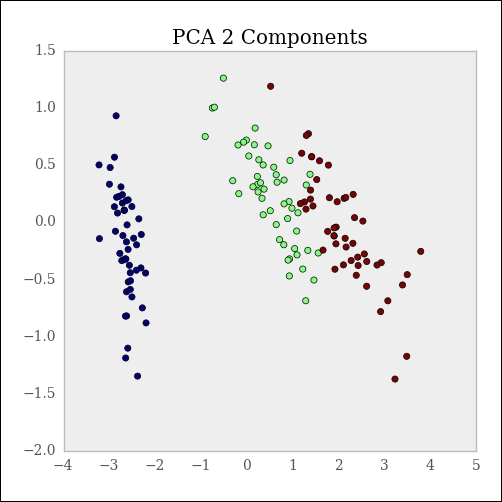The separability of the classes remain even after reducing the number of dimensionality by two.

We can see how much of the variance is represented by the two components that remain:

```>>> pca.explained_variance_ratio_.sum()
0.9776
```

### There's more...

The PCA object can also be created with the amount of explained variance in mind from the start. For example, if we want to be able to explain at least 98 percent of the variance, the PCA object will be created as follows:

```>>> pca = decomposition.PCA(n_components=.98)
>>> iris_X_prime = pca.fit(iris_X)
>>> pca.explained_variance_ratio_.sum()
1.0
```

Since we wanted to explain variance slightly more than the two component examples, a third was included.

## Using factor analysis for decomposition

Factor analysis is another technique we can use to reduce dimensionality. However, factor analysis makes assumptions and PCA does not. The basic assumption is that there are implicit features responsible for the features of the dataset.

This recipe will boil down to the explicit features from our samples in an attempt to understand the independent variables as much as the dependent variables.

To compare PCA and factor analysis, let's use the `iris` dataset again, but we'll first need to load the factor analysis class:

```>>> from sklearn.decomposition import FactorAnalysis
```

### How to do it...

From a programming perspective, factor analysis isn't much different from PCA:

```>>> fa = FactorAnalysis(n_components=2)
>>> iris_two_dim = fa.fit_transform(iris.data)
>>> iris_two_dim[:5]
array([[-1.33125848,  0.55846779],
[-1.33914102, -0.00509715],
[-1.40258715, -0.307983  ],
[-1.29839497, -0.71854288],
[-1.33587575,  0.36533259]])
```

### Tip

You can download the example code files for all Packt books you have purchased from your account at http://www.packtpub.com. If you purchased this book elsewhere, you can visit http://www.packtpub.com/support and register to have the files e-mailed directly to you

Compare the following plot to the plot in the last section: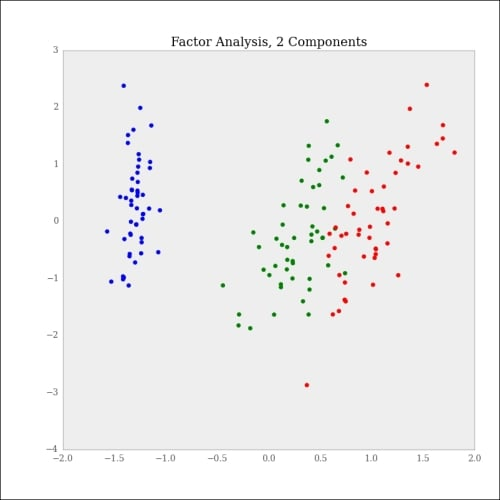Since factor analysis is a probabilistic transform, we can examine different aspects such as the log likelihood of the observations under the model, and better still, compare the log likelihoods across models.

Factor analysis is not without flaws. The reason is that you're not fitting a model to predict an outcome, you're fitting a model as a preparation step. This isn't a bad thing per se, but errors here compound when training the actual model.

### How it works...

Factor analysis is similar to PCA, which was covered previously. However, there is an important distinction to be made. PCA is a linear transformation of the data to a different space where the first component "explains" the variance of the data, and each subsequent component is orthogonal to the first component.

For example, you can think of PCA as taking a dataset of N dimensions and going down to some space of M dimensions, where M < N.

Factor analysis, on the other hand, works under the assumption that there are only M important features and a linear combination of these features (plus noise) creates the dataset in N dimensions. To put it another way, you don't do regression on an outcome variable, you do regression on the features to determine the latent factors of the dataset.

## Kernel PCA for nonlinear dimensionality reduction

Most of the techniques in statistics are linear by nature, so in order to capture nonlinearity, we might need to apply some transformation. PCA is, of course, a linear transformation. In this recipe, we'll look at applying nonlinear transformations, and then apply PCA for dimensionality reduction.

Life would be so easy if data was always linearly separable, but unfortunately it's not. Kernel PCA can help to circumvent this issue. Data is first run through the kernel function that projects the data onto a different space; then PCA is performed.

To familiarize yourself with the kernel functions, it will be a good exercise to think of how to generate data that is separable by the kernel functions available in the kernel PCA. Here, we'll do that with the cosine kernel. This recipe will have a bit more theory than the previous recipes.

### How to do it...

The cosine kernel works by comparing the angle between two samples represented in the feature space. It is useful when the magnitude of the vector perturbs the typical distance measure used to compare samples.

As a reminder, the cosine between two vectors is given by the following: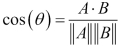This means that the cosine between A and B is the dot product of the two vectors normalized by the product of the individual norms. The magnitude of vectors A and B have no influence on this calculation.

So, let's generate some data and see how useful it is. First, we'll imagine there are two different underlying processes; we'll call them A and B:

```>>> import numpy as np
>>> A1_mean = [1, 1]
>>> A1_cov = [[2, .99], [1, 1]]
>>> A1 = np.random.multivariate_normal(A1_mean, A1_cov, 50)

>>> A2_mean = [5, 5]
>>> A2_cov = [[2, .99], [1, 1]]
>>> A2 = np.random.multivariate_normal(A2_mean, A2_cov, 50)

>>> A = np.vstack((A1, A2))

>>> B_mean = [5, 0]
>>> B_cov = [[.5, -1], [-.9, .5]]
>>> B = np.random.multivariate_normal(B_mean, B_cov, 100)
```

Once plotted, it will look like the following: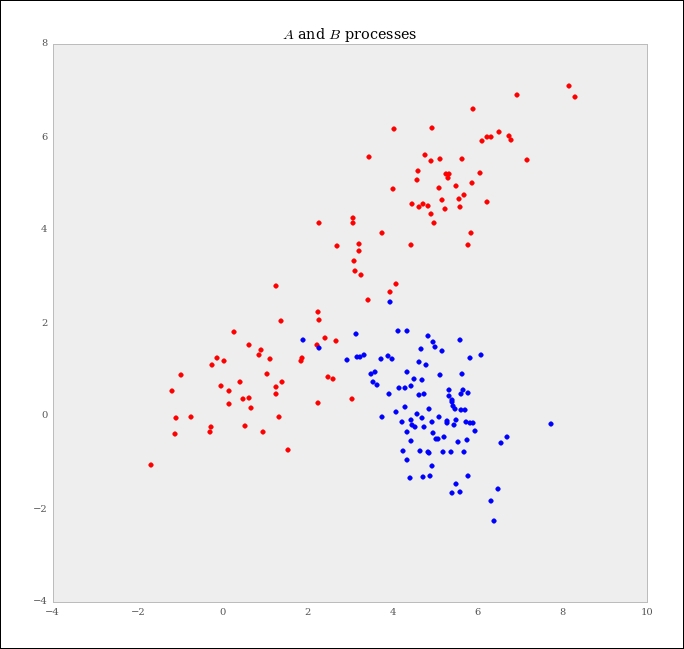By visual inspection, it seems that the two classes are from different processes, but separating them in one slice might be difficult. So, we'll use the kernel PCA with the cosine kernel discussed earlier:

```>>> kpca = decomposition.KernelPCA(kernel='cosine', n_components=1)
>>> AB = np.vstack((A, B))
>>> AB_transformed = kpca.fit_transform(AB)
```

Visualized in one dimension after the kernel PCA, the dataset looks like the following: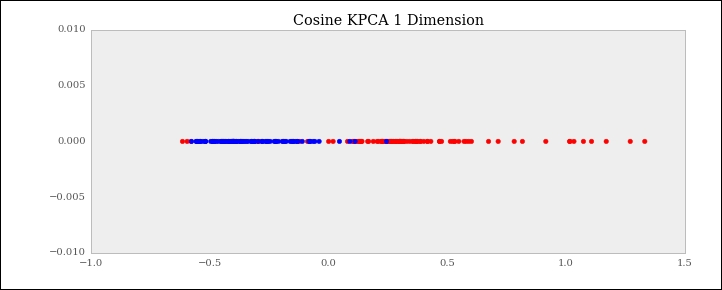Contrast this with PCA without a kernel: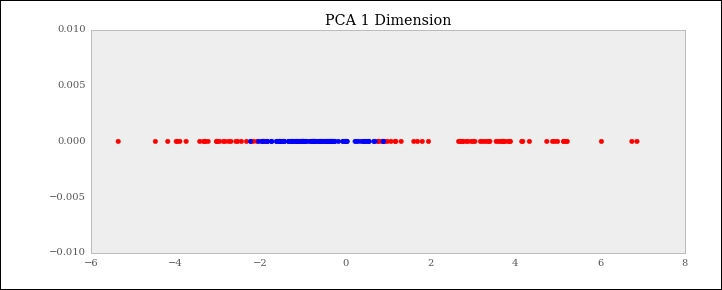Clearly, the kernel PCA does a much better job.

### How it works...

There are several different kernels available as well as the cosine kernel. You can even write your own kernel function. The available kernels are:

• `poly` (polynomial)

• `rbf` (radial basis function)

• `sigmoid`

• `cosine`

• `precomputed`

There are also options contingent of the kernel choice. For example, the degree argument will specify the degree for the `poly`, `rbf`, and `sigmoid` kernels; also, gamma will affect the `rbf` or `poly` kernels.

The recipe on SVM will cover the `rbf` kernel function in more detail.

A word of caution: kernel methods are great to create separability, but they can also cause overfitting if used without care.

## Using truncated SVD to reduce dimensionality

Truncated Singular Value Decomposition (SVD) is a matrix factorization technique that factors a matrix M into the three matrices U, Î£, and V. This is very similar to PCA, excepting that the factorization for SVD is done on the data matrix, whereas for PCA, the factorization is done on the covariance matrix. Typically, SVD is used under the hood to find the principle components of a matrix.

Truncated SVD is different from regular SVDs in that it produces a factorization where the number of columns is equal to the specified truncation. For example, given an n x n matrix, SVD will produce matrices with n columns, whereas truncated SVD will produce matrices with the specified number of columns. This is how the dimensionality is reduced.

Here, we'll again use the `iris` dataset so that you can compare this outcome against the PCA outcome:

```>>> from sklearn.datasets import load_iris
>>> iris_data = iris.data
>>> iris_target = iris.target
```

### How to do it...

This object follows the same form as the other objects we've used. First, we'll import the required object, then we'll fit the model and examine the results:

```>>> from sklearn.decomposition import TruncatedSVD
>>> svd = TruncatedSVD(2)
>>> iris_transformed = svd.fit_transform(iris_data)
>>> iris_data[:5]
array([[ 5.1,  3.5,  1.4,  0.2],
[ 4.9,  3. ,  1.4,  0.2],
[ 4.7,  3.2,  1.3,  0.2],
[ 4.6,  3.1,  1.5,  0.2],
[ 5. ,  3.6,  1.4,  0.2]])

>>> iris_transformed[:5]
array([[ 5.91220352, -2.30344211],
[ 5.57207573, -1.97383104],
[ 5.4464847 , -2.09653267],
[ 5.43601924, -1.87168085],
[ 5.87506555, -2.32934799]])
```

The output will look like the following: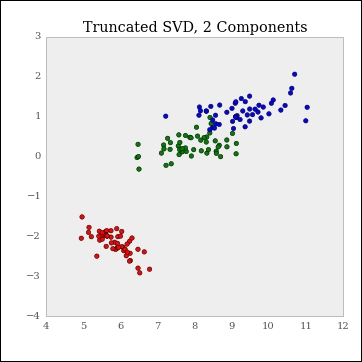### How it works...

Now that we've walked through how `TruncatedSVD` is performed in scikit-learn, let's look at how we can use only `scipy`, and learn a bit in the process.

First, we need to use `linalg` of `scipy` to perform SVD:

```>>> from scipy.linalg import svd
>>> D = np.array([[1, 2], [1, 3], [1, 4]])
>>> D
array([[1, 2],
[1, 3],
[1, 4]])

>>> U, S, V = svd(D, full_matrices=False)
>>> U.shape, S.shape, V.shape
((3, 2), (2,), (2, 2))
```

We can reconstruct the original matrix D to confirm U, S, and V as a decomposition:

```>>> np.dot(U.dot(np.diag(S)), V)
array([[1, 2],
[1, 3],
[1, 4]])
```

The matrix that is actually returned by `TruncatedSVD` is the dot product of the U and S matrices.

If we want to simulate the truncation, we will drop the smallest singular values and the corresponding column vectors of U. So, if we want a single component here, we do the following:

```>>> new_S = S
>>> new_U = U[:, 0]
>>> new_U.dot(new_S)
array([-2.20719466, -3.16170819, -4.11622173])
```

In general, if we want to truncate to some dimensionality, for example, t, we drop N-t singular values.

### There's more...

`TruncatedSVD` has a few miscellaneous things that are worth noting with respect to the method.

#### Sign flipping

There's a "gotcha" with truncated SVDs. Depending on the state of the random number generator, successive fittings of `TruncatedSVD` can flip the signs of the output. In order to avoid this, it's advisable to fit `TruncatedSVD` once, and then use transforms from then on. Another good reason for Pipelines!

To carry this out, do the following:

```>>> tsvd = TruncatedSVD(2)
>>> tsvd.fit(iris_data)
>>> tsvd.transform(iris_data)
```

#### Sparse matrices

One advantage of `TruncatedSVD` over PCA is that `TruncatedSVD` can operate on sparse matrices while PCA cannot. This is due to the fact that the covariance matrix must be computed for PCA, which requires operating on the entire matrix.

## Decomposition to classify with DictionaryLearning

In this recipe, we'll show how a decomposition method can actually be used for classification. `DictionaryLearning` attempts to take a dataset and transform it into a sparse representation.

With `DictionaryLearning`, the idea is that the features are a basis for the resulting datasets. In an effort to keep this recipe short, I'll assume you have `idis_data` and `iris_target` ready to go.

### How to do it...

First, import `DictionaryLearning`:

```>>> from sklearn.decomposition import DictionaryLearning
```

Next, use three components to represent the three species of `iris`:

```>>> dl = DictionaryLearning(3)
```

Then transform every other data point so that we can test the classifier on the resulting data points after the learner is trained:

```>>> transformed = dl.fit_transform(iris_data[::2])
>>> transformed[:5]
array([[ 0.        ,  6.34476574,  0.        ],
[ 0.        ,  5.83576461,  0.        ],
[ 0.        ,  6.32038375,  0.        ],
[ 0.        ,  5.89318572,  0.        ],
[ 0.        ,  5.45222715,  0.        ]])
```

We can visualize the output. Notice how each value is sited on the x, y, or z axis along with the other values and 0; this is called sparseness.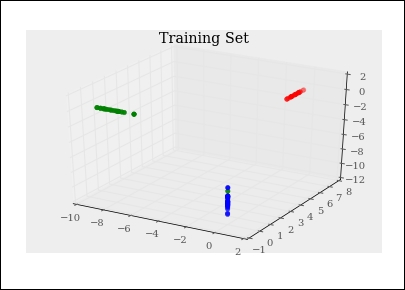If you look closely, you can see there was some training error. One of the classes was misclassified. Only being wrong once isn't a big deal, though.

Next, let's fit (not `fit_transform`) the testing set:

```>>> transformed = dl.transform(iris_data[1::2])
```

The following screenshot shows its performance: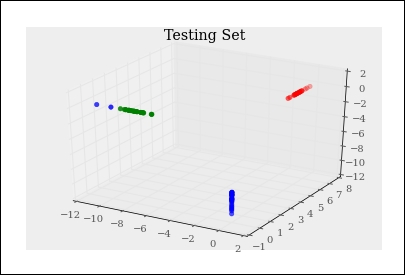Notice again that there was some error in the classification. If you remember some of the other visualizations, the blue and green classes were the two classes that often appeared close together.

### How it works...

`DictionaryLearning` has a background in signal processing and neurology. The idea is that only few features can be active at any given time. Therefore, `DictionaryLearning` attempts to find a suitable representation for the underlying data, given the constraint that most of the features should be `0`.

## Putting it all together with Pipelines

Now that we've used Pipelines and data transformation techniques, we'll walk through a more complicated example that combines several of the previous recipes into a pipeline.

In this section, we'll show off some more of Pipeline's power. When we used it earlier to impute missing values, it was only a quick taste; we'll chain together multiple preprocessing steps to show how Pipelines can remove extra work.

Let's briefly load the `iris` dataset and seed it with some missing values:

```>>> from sklearn.datasets import load_iris
>>> import numpy as np

>>> iris_data = iris.data

>>> mask = np.random.binomial(1, .25, iris_data.shape).astype(bool)
>>> iris_data[:5]
array([[ 5.1,  3.5,  1.4,  nan],
[ nan,  3. ,  1.4,  0.2],
[ 4.7,  3.2,  1.3,  0.2],
[ 4.6,  3.1,  1.5,  0.2],
[ 5. ,  3.6,  nan,  0.2]])
```

### How to do it...

The goal of this chapter is to first impute the missing values of `iris_data`, and then perform PCA on the corrected dataset. You can imagine (and we'll do it later) that this workflow might need to be split between a training dataset and a holdout set; Pipelines will make this easier, but first we need to take a baby step.

```>>> from sklearn import pipeline, preprocessing, decomposition
```

Next, create the imputer and PCA classes:

```>>> pca = decomposition.PCA()
>>> imputer = preprocessing.Imputer()
```

Now that we have the classes we need, we can load them into Pipeline:

```>>> pipe = pipeline.Pipeline([('imputer', imputer), ('pca', pca)])
>>> iris_data_transformed = pipe.fit_transform(iris_data)
>>> iris_data_transformed[:5]
array([[ -2.42e+00,  -3.59e-01,  -6.88e-01,  -3.49e-01],
[ -2.44e+00,  -6.94e-01,   3.27e-01,   4.87e-01],
[ -2.94e+00,   2.45e-01,  -1.85e-03,   4.37e-02],
[ -2.79e+00,   4.29e-01,  -8.05e-03,   9.65e-02],
[ -6.46e-01,   8.87e-01,   7.54e-01,  -5.19e-01]])
```

This takes a lot more management if we use separate steps. Instead of each step requiring a fit transform, this step is performed only once. Not to mention that we only have to keep track of one object!

### How it works...

Hopefully it was obvious, but each step in Pipeline is passed to a Pipeline object via a list of tuples, with the first element getting the name and the second getting the actual object.

Under the hood, these steps are looped through when a method such as `fit_transform` is called on the Pipeline object.

This said, there are quick and dirty ways to create Pipeline, much in the same way there was a quick way to perform scaling, though we can use `StandardScaler` if we want more power. The `pipeline` function will automatically create the names for the Pipeline objects:

```>>> pipe2 = pipeline.make_pipeline(imputer, pca)
>>> pipe2.steps
[('imputer', Imputer(axis=0, copy=True, missing_values='NaN', strategy='mean', verbose=0)),
('pca', PCA(copy=True, n_components=None, whiten=False))]
```

This is the same object that was created in the more verbose method:

```>>> iris_data_transformed2 = pipe2.fit_transform(iris_data)
>>> iris_data_transformed2[:5]
array([[ -2.42e+00,  -3.59e-01,  -6.88e-01,  -3.49e-01],
[ -2.44e+00,  -6.94e-01,   3.27e-01,   4.87e-01],
[ -2.94e+00,   2.45e-01,  -1.85e-03,   4.37e-02],
[ -2.79e+00,   4.29e-01,  -8.05e-03,   9.65e-02],
[ -6.46e-01,   8.87e-01,   7.54e-01,  -5.19e-01]])
```

### There's more...

We just walked through Pipelines at a very high level, but it's unlikely that we will want to apply the base transformation. Therefore, the attributes of each object in Pipeline can be accessed from a `set_params` method, where the parameter follows the `<parameter's_name>__<parameter's_parameter>` convention. For example, let's change the `pca` object to use two components:

```>>> pipe2.set_params(pca_n_components=2)
Pipeline(steps=[('imputer', Imputer(axis=0, copy=True,
missing_values='NaN', strategy='mean', verbose=0)),
('pca', PCA(copy=True, n_components=2, whiten=False))])
```

### Tip

The `__` notation is pronounced as dunder in the Python community.

Notice `n_components=2` in the preceding output. Just as a test, we can output the same transformation we already did twice, and the output will be an N x 2 matrix:

```>>> iris_data_transformed3 = pipe2.fit_transform(iris_data)
>>> iris_data_transformed3[:5]
array([[-2.42, -0.36],
[-2.44, -0.69],
[-2.94,  0.24],
[-2.79,  0.43],
[-0.65,  0.89]])
```

## Using Gaussian processes for regression

In this recipe, we'll use the Gaussian process for regression. In the linear models section, we saw how representing prior information on the coefficients was possible using Bayesian Ridge Regression.

With a Gaussian process, it's about the variance and not the mean. However, with a Gaussian process, we assume the mean is 0, so it's the covariance function we'll need to specify.

The basic setup is similar to how a prior can be put on the coefficients in a typical regression problem. With a GP, a prior can be put on the functional form of the data, and it's the covariance between the data points that is used to model the data, and therefore, must be fit from the data.

So, let's use some regression data and walkthrough how Gaussian processes work in scikit-learn:

```>>> from sklearn.datasets import load_boston

>>> boston_X = boston.data
>>> boston_y = boston.target

>>> train_set = np.random.choice([True, False], len(boston_y),
p=[.75, .25])
```

### How to do itâ€¦

Now that we have the data, we'll create a scikit-learn `GaussianProcess` object. By default, it uses a constant regression function and squared exponential correlation, which is one of the more common choices:

```>>> from sklearn.gaussian_process import GaussianProcess
>>> gp = GaussianProcess()
>>> gp.fit(boston_X[train_set], boston_y[train_set])
GaussianProcess(beta0=None, corr=<function squared_exponential
at 0x110809488>, normalize=True,
nugget=array(2.220446049250313e-15),
optimizer='fmin_cobyla', random_start=1,
random_state=<mtrand.RandomState object
at 0x10b9b58b8>, regr=<function constant
at 0x1108090c8>, storage_mode='full',
theta0=array([[ 0.1]]), thetaL=None, thetaU=None,
verbose=False)
```

That's a formidable object definition. The following are a couple of things to point out:

• `beta0`: This is the regression weight. This defaults in a way such that MLE is used for estimation.

• `corr`: This is the correlation function. There are several built-in correlation functions. We'll look at more of them in the following How it works... section.

• `regr`: This is the constant regression function.

• `nugget`: This is the regularization parameter. It defaults to a very small number. You can either pass one value to be used for each data point or a single value that needs to be applied uniformly.

• `normalize`: This defaults to `True`, and it will center and scale the features. This would be scale is R.

Okay, so now that we fit the object, let's look at it's performance against the test object:

```>>> test_preds = gp.predict(boston_X[~train_set])
```

Let's plot the predicted values versus the actual values; then, because we're doing regression, it's probably a good idea to look at plotted residuals and a histogram of the residuals:

```>>> from matplotlib import pyplot as plt
>>> f, ax = plt.subplots(figsize=(10, 7), nrows=3)
>>> f.tight_layout()

>>> ax.plot(range(len(test_preds)), test_preds,
label='Predicted Values');
>>> ax.plot(range(len(test_preds)), boston_y[~train_set],
label='Actual Values');
>>> ax.set_title("Predicted vs Actuals")
>>> ax.legend(loc='best')

>>> ax.plot(range(len(test_preds)),
test_preds - boston_y[~train_set]);
>>> ax.set_title("Plotted Residuals")

>>> ax.hist(test_preds - boston_y[~train_set]);
>>> ax.set_title("Histogram of Residuals")
```

The output is as follows: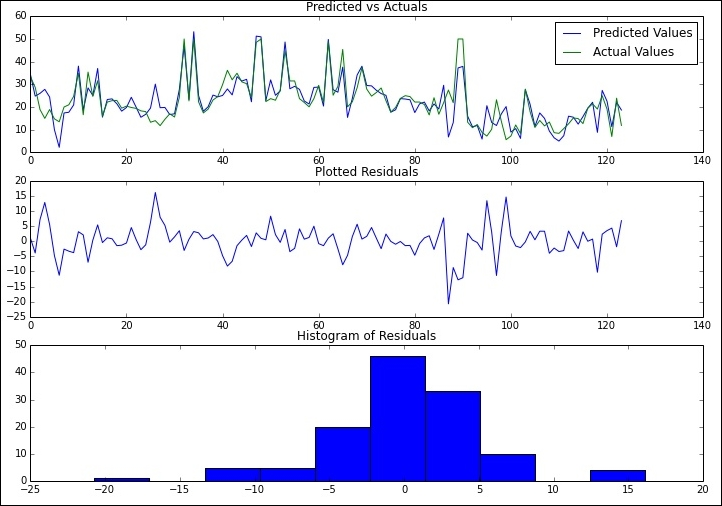### How it worksâ€¦

Now that we've worked through a very quick example, let's look a little more at what some of the parameters do and how we can tune them based on the model we're trying to fit.

First, let's try to understand what's going on with the `corr` function. This function describes the relationship between the different pairs of X. The following five different correlation functions are offered by scikit-learn:

• `absolute_exponential`

• `squared_exponential`

• `generalized_exponential`

• `cubic`

• `linear`

For example, the squared exponential has the following form: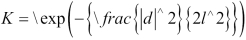Linear, on the other hand, is just the dot product of the two points in question: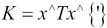Another parameter of interest is `theta0`. This represents the starting point in the estimation of the the parameters.

Once we have an estimation of K and the mean, the process is fully specified due to it being a Gaussian process; emphasis is put on Gaussian, a reason it's so popular for general machine learning work.

Let's use a different `regr` function, apply a different `theta0`, and look at how the predictions differ:

```>>> gp = GaussianProcess(regr='linear', theta0=5e-1)
>>> gp.fit(boston_X[train_set], boston_y[train_set]);
>>> linear_preds = gp.predict(boston_X[~train_set])
>>> f, ax = plt.subplots(figsize=(7, 5))
```

Let's have a look at the fit:

```>>> f.tight_layout()

>>> ax.hist(test_preds - boston_y[~train_set],
label='Residuals Original', color='b', alpha=.5);
>>> ax.hist(linear_preds - boston_y[~train_set],
label='Residuals Linear', color='r', alpha=.5);
>>> ax.set_title("Residuals")
>>> ax.legend(loc='best')
```

The following is the output: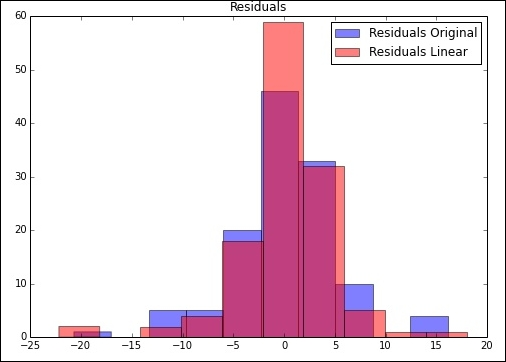Clearly, the second model's predictions are slightly better for the most part. If we want to sum this up, we can look at the MSE of the predictions:

```>>> np.power(test_preds - boston_y[~train_set], 2).mean()
26.254844099612455
>>> np.power(linear_preds - boston_y[~train_set], 2).mean()
21.938924337056068
```

### There's moreâ€¦

We might want to understand the uncertainty in our estimates. When we make the predictions, if we pass the `eval_MSE` argument as `True`, we'll get MSE and the predicted values. From a mechanics standpoint, a tuple of predictions and MSE is returned:

```>>> test_preds, MSE = gp.predict(boston_X[~train_set], eval_MSE=True)
>>> MSE[:5]
array([ 11.95314572,   8.48397825,   6.0287539 ,  29.20844347,
0.36427829])
```

So, now that we have errors in the estimates (unfortunately), let's plot the first few to get an indication of accuracy:

```>>> f, ax = plt.subplots(figsize=(7, 5))

>>> n = 20
>>> rng = range(n)
>>> ax.scatter(rng, test_preds[:n])
>>> ax.errorbar(rng, test_preds[:n], yerr=1.96*MSE[:n])

>>> ax.set_title("Predictions with Error Bars")

>>> ax.set_xlim((-1, 21));
```

The following is the output: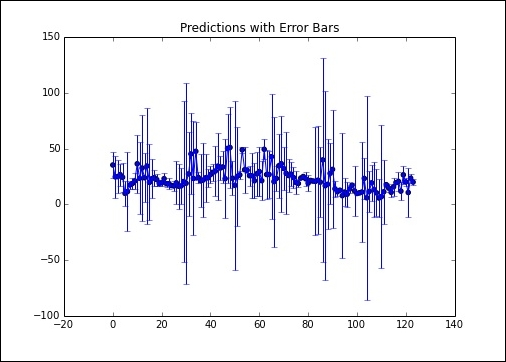As you can see, there's quite a bit of variance in the estimates for a lot of these points. On the other hand, our overall error wasn't too bad.

## Defining the Gaussian process object directly

We just touched the surface of Gaussian processes. In this recipe, we'll look at how we can directly access the Gaussian process object with the correlation function we want.

Within the `gaussian_process` module, there is direct access to many of the correlation functions or regression functions. This means that instead of creating the `GaussianProcess` object, we can just create this object through a function. If you're more familiar with object-oriented code, this is basically a class method at the module level.

In this chapter, we'll march through most of the functions and show their results on example data. Do not stop at these examples if you want to get more familiar with the behavior of the various covariate functions. Hopefully, you're still using IPython (or the notebook).

Since this doesn't expose anything thing new mathematically, we'll just show how to do it.

### How to do itâ€¦

First, we'll import some basic regression data:

```>>> from sklearn.datasets import make_regression
>>> X, y = make_regression(1000, 1, 1)
>>> from sklearn.gaussian_process import regression_models
```

First up is the constant correlation function. This will comprise a constant and more for completeness:

```>>> regression_models.constant(X)[:5]
array([[ 1.],
[ 1.],
[ 1.],
[ 1.],
[ 1.]])
```

Another option is the squared exponential correlation function. This is also the default for the `GaussianProcess` class:

```>>> regression_models.linear(X)[:1]
array([[ 1., 0.38833572]])

array([[ 1., 0.38833572, 0.15080463]])
```

### How it worksâ€¦

Now that we have the regression function, we can feed it directly into the `GaussianProcess` object. The default is the constant regression function, but we can just as easily pass it in a linear model or a quadratic model.

## Using stochastic gradient descent for regression

In this recipe, we'll get our first taste of stochastic gradient descent. We'll use it for regression here, but for the next recipe, we'll use it for classification.

Stochastic Gradient Descent (SGD) is often an unsung hero in machine learning. Underneath many algorithms, there is SGD doing the work. It's popular due to its simplicity and speedâ€”these are both very good things to have when dealing with a lot of data.

The other nice thing about SGD is that while it's at the core of many ML algorithms computationally, it does so because it easily describes the process. At the end of the day, we apply some transformation on the data, and then we fit our data to the model with some loss function.

### How to do itâ€¦

If SGD is good on large datasets, we should probably test it on a fairly large dataset:

```>>> from sklearn import datasets
>>> X, y = datasets.make_regression(int(1e6))
# Just in case the 1e6 throws you off.
>>> print "{:,}".format(int(1e6))
1,000,000
```

It's probably worth gaining some intuition about the composition and size of the object. Thankfully, we're dealing with NumPy arrays, so we can just access `nbytes`. The built-in Python way to access the object size doesn't work for NumPy arrays. This output be system dependent, so you may not get the same results:

```>>> print "{:,}".format(X.nbytes)
800,000,000
```

To get some human perspective, we can convert `nbytes` to megabytes. There are roughly 1 million bytes in an MB:

```>>> X.nbytes / 1e6
800.0
```

So, the number of bytes per data point is:

```>>> X.nbytes / (X.shape*X.shape)
8
```

Well, isn't that tidy, and fairly tangential, for what we're trying to accomplish; however, it's worth knowing how to get the size of the objects you're dealing with.

So, now that we have the data, we can simply fit a `SGDRegressor` model:

```>>> from sklearn import linear_model
>>> sgd = linear_model.SGDRegressor()
>>> train = np.random.choice([True, False], size=len(y), p=[.75, .25])
>>> sgd.fit(X[train], y[train])
SGDRegressor(alpha=0.0001, epsilon=0.1, eta0=0.01,
fit_intercept=True, l1_ratio=0.15,
learning_rate='invscaling', loss='squared_loss',
n_iter=5, penalty='l2', power_t=0.25, random_state=None,
shuffle=False, verbose=0, warm_start=False)
```

So, we have another "beefy" object. The main thing to know now is that our loss function is `squared_loss`, which is the same thing that occurs during linear regression. Also worth noting is that shuffle will generate a random shuffle of the data. This is useful if you want to break a potentially spurious correlation. With `fit_intercept`, scikit-learn will automatically include a column of ones. If you like to see more through the output of the fitting, set `verbose` to `1`.

We can then predict, as we previously have, using scikit-learn's consistent API: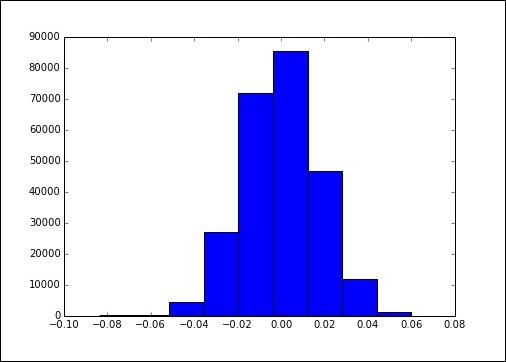You can see we actually got a really good fit. There is barely any variation and the histogram has a nice normal look.

### How it worksâ€¦

Clearly, the fake dataset we used wasn't too bad, but you can imagine datasets with larger magnitudes. For example, if you worked in Wall Street on any given day, there might be two billion transactions on any given exchange in a market. Now, imagine that you have a week's or year's data. Running in-core algorithms does not work with huge volumes of data.

The reason this is normally difficult is that to do standard gradient descent, we're required to calculate the gradient at every step. The gradient has the standard definition from any third calculus course.

The gist of the algorithm is that at each step we calculate a new set of coefficients and update this by a learning rate and the outcome of the objective function.

In pseudo code, this might look like the following:

```>>> while not_converged:
```

The relevant variables are as follows:

• `w`: This is the coefficient matrix.

• `learning_rate`: This shows how big a step to take at each iteration. This might be important to tune if you aren't getting a good convergence.

• `gradient`: This is the matrix of second derivatives.

• `cost`: This is the squared error for regression. We'll see later that this cost function can be adapted to work with classification tasks. This flexibility is one thing that makes SGD so useful.

This will not be so bad, except for the fact that the gradient function is expensive. As the vector of coefficients gets larger, calculating the gradient becomes very expensive. For each update step, we need to calculate a new weight for every point in the data, and then update.

The stochastic gradient descent works slightly differently; instead of the previous definition for batch gradient descent, we'll update the parameter with each new data point. This data point is picked at random, and hence the name stochastic gradient descent.